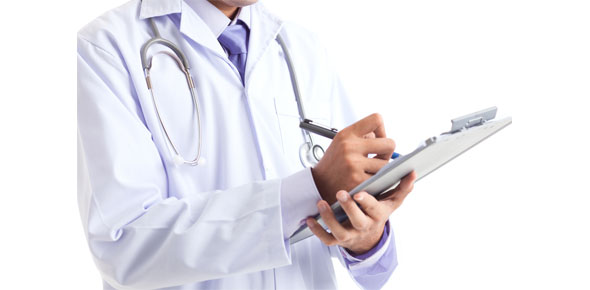# Ks3 Science Diagnostic Test

30 Questions | Total Attempts: 2567SettingsKS3 Science Diagnostic Test, suitable for pupils in Yr8 and Yr9.

• 1.
What do animal and plant cells have in common?
• A.

Cell membrane, nucleus

• B.

Cell membrane, cell wall

• C.

Vacuole, nucleus

• D.

Cell wall, nucleus

• E.

Chloroplasts and vacuole

• 2.
What shape best describes a red blood cell?
• A.

Spherical

• B.

Square

• C.

Oval

• D.

Biconcave

• E.

Rectangular

• 3.
What are the tubes called that carry water to the leaf?
• A.

Phloem

• B.

Xylem

• C.

Stem

• D.

Root

• E.

Leaf

• 4.
Which organs turns waste products into urine?
• A.

• B.

Lungs

• C.

Kidney

• D.

Heart

• E.

Liver

• 5.
Where does photosynthesis take place?
• A.

The flower

• B.

The root

• C.

The stem

• D.

The leaf

• E.

The fruit

• 6.
What is a cell membrane for?
• A.

Controlling the activities of the cell

• B.

It is where chemical reaction take place

• C.

Controlling what passes in and out of the cells

• D.

To contain genes

• E.

To keep the leaf in shape

• 7.
Which statement is true for cytoplasm?
• A.

Controls what enters and leaves the cell

• B.

Keep the shape of the cell stops the cell from bursting

• C.

Controls what happen in the cell

• D.

Where the cell's chemical reactions take place

• E.

Helps to absorb water

• 8.
What is meant by menstruation?
• A.

An egg is released

• B.

Blood from the uterus wall is lost

• C.

Fertilisation takes place

• D.

The lining of the uterus starts to grow again.

• E.

Supplying protein to the baby & the pregnant lady

• 9.
What is meant by ovulation?
• A.

An egg is released

• B.

Blood from the uterus wall is lost

• C.

Fertilisation takes place

• D.

The lining of the uterus starts to grow again.

• E.

Supplying protein to the baby & the pregnant lady

• 10.
Tobacco contains a very poisonous substance. Which one of the following is correct?
• A.

Benzene

• B.

Chlorophyll

• C.

Nicotine

• D.

Calcium

• E.

Mercury

• 11.
Which two properties about hydrogen gas are correct?(Tick 2 options)
• A.

Hydrogen does not dissolve in water.

• B.

Hydrogen dissolves in water.

• C.

Hydrogen has a lower density than air.

• D.

Hydrogen is more dense than air.

• E.

Hydrogen does not dissolve in water & is more dense than air.

• 12.
What is produced when hydrogen burns in air?
• A.

Hydrogen Oxide or Water.

• B.

Nitrogen

• C.

Oxygen

• D.

Carbon Di Oxide

• E.

Carbon Mono Oxide

• 13.
Which three elements are combined in ethanol?
• A.

Carbon, hydrogen & oxygen

• B.

Sulphur, nitrogen & hydrogen

• C.

Calcium, potassium & chlorine

• D.

Bromine, benzene & silver

• E.

Sulphur, copper & iodine

• 14.
What do we call a mixture of sodium chloride and water?
• A.

Solvent

• B.

Solute

• C.

Solution

• D.

Soluble

• E.

Insoluble

• 15.
Which gas, found in the air, dissolves in rain water to form acidic rain?
• A.

Oxygen

• B.

Nitrogen

• C.

Carbon dioxide

• D.

Neon

• E.

Argon

• 16.
How does carbon dioxide cause global warming?
• A.

The gas trap the heat reaching the earth from the sun

• B.

It makes acid rain

• C.

It destroys the ozone layer

• D.

It attacks the chalk and limestone

• E.

It reduces the temparature of the air

• 17.
Tick the correct statements below
• A.

Copper sulphate is insoluable in water

• B.

Sugar is more soluable in hot water than cold water

• C.

Most solutes are more soluble at high tempaeratures

• D.

Water is a solvent or all solids

• E.

When no more solute can dissolve in a solution it has formed a saturated solution

• 18.
Ice is an example of which state of matter?
• A.

Gas

• B.

Liquid

• C.

Solid

• D.

Steam

• E.

Ion

• 19.
What  happens to particles when they are heated?
• A.

They move less

• B.

They get bigger

• C.

They move around more

• D.

They get smaller

• E.

They become stabilized

• 20.
Which particles are found in shells around a nucleus?
• A.

Protons & neutrons

• B.

Protons & electrons

• C.

Electrons only

• D.

Neutrons only

• E.

Masons

• 21.
How far will a bus travel in 5 hours if its speed is 60 km/hour?
• A.

12 km

• B.

24 km

• C.

300 km

• D.

120 km

• E.

700 km

• 22.
A sloping straight line on a distance-time graph tells us that the object is:
• A.

Accelerating

• B.

Going up hill

• C.

Going down hill

• D.

Decelerating

• E.

Moving at a constant speed

• 23.
Which of the following will happen if balanced forces are applied to an object?
• A.

It will accelerate

• B.

It will decelerate

• C.

It may travel at a constant speed

• D.

It will change direction

• E.

It will move slightly

• 24.
Friction between the surfaces can be increased by :
• A.

Polishing the surfaces

• B.

Roughening the surfaces

• C.

Using a lubricant

• D.

• E.

Making streamlined

• 25.
What pressure is created when a force of 64 N is applied over an area of 4 metre square?
• A.

256 Pa

• B.

16 Nm

• C.

16 Pa

• D.

256 Nm

• E.

300 Pa

Related TopicsBack to top